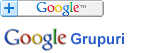### Căutare în Webster - Dicționarul explicativ al limbii engleze

 Cuvânt
Pentru căutare rapidă introduceți minim 3 litere.

## FUNCTION - Definiția din dicționar

Notă: Puteţi căuta fiecare cuvânt din cadrul definiţiei printr-un simplu click pe cuvântul dorit.

Func"tion (?), n. [L. functio, fr. fungi to perform, execute, akin to Skr. bhuj to enjoy, have the use of: cf. F. fonction. Cf. Defunct.] 1. In the function of his public calling.” Swift.
[1913 Webster]

2. (Physiol.)
[1913 Webster]

3.
[1913 Webster]

As the mind opens, and its functions spread. Pope.
[1913 Webster]

4.
[1913 Webster]

[1913 Webster]

regal functions.
Macaulay.
[1913 Webster]

5. (Math.)
[1913 Webster]

6. (Eccl.)

Every solemnfunction' performed with the requirements of the liturgy. Card. Wiseman.
[Webster 1913 Suppl.]

7.

This function, which is our chief social event. W. D. Howells.
[Webster 1913 Suppl.]

Algebraic function, a quantity whose connection with the variable is expressed by an equation that involves only the algebraic operations of addition, subtraction, multiplication, division, raising to a given power, and extracting a given root; -- opposed to transcendental function. -- Arbitrary function. See under Arbitrary. -- Calculus of functions. See under Calculus. -- Carnot's function (Thermo-dynamics), a relation between the amount of heat given off by a source of heat, and the work which can be done by it. It is approximately equal to the mechanical equivalent of the thermal unit divided by the number expressing the temperature in degrees of the air thermometer, reckoned from its zero of expansion. -- Circular functions. See Inverse trigonometrical functions (below). -- Continuous function, a quantity that has no interruption in the continuity of its real values, as the variable changes between any specified limits. -- Discontinuous function. See under Discontinuous. -- Elliptic functions, a large and important class of functions, so called because one of the forms expresses the relation of the arc of an ellipse to the straight lines connected therewith. -- Explicit function, a quantity directly expressed in terms of the independently varying quantity; thus, in the equations y = 6x2, y = 10 -x3, the quantity y is an explicit function of x. -- Implicit function, a quantity whose relation to the variable is expressed indirectly by an equation; thus, y in the equation x2 + y2 = 100 is an implicit function of x. -- Inverse trigonometrical functions, or Circular functions, the lengths of arcs relative to the sines, tangents, etc. Thus, AB is the arc whose sine is BD, and (if the length of BD is x) is written sin -1x, and so of the other lines. See Trigonometrical function (below). Other transcendental functions are the exponential functions, the elliptic functions, the gamma functions, the theta functions, etc. -- One-valued function, a quantity that has one, and only one, value for each value of the variable. -- Transcendental functions, a quantity whose connection with the variable cannot be expressed by algebraic operations; thus, y in the equation y = 10x is a transcendental function of x. See Algebraic function (above). -- Trigonometrical function, a quantity whose relation to the variable is the same as that of a certain straight line drawn in a circle whose radius is unity, to the length of a corresponding are of the circle. Let AB be an arc in a circle, whose radius OA is unity let AC be a quadrant, and let OC, DB, and AF be drawnpependicular to OA, and EB and CG parallel to OA, and let OB be produced to G and F. E Then BD is the sine of the arc AB; OD or EB is the cosine, AF is the tangent, CG is the cotangent, OF is the secant OG is the cosecant, AD is the versed sine, and CE is the coversed sine of the are AB. If the length of AB be represented by x (OA being unity) then the lengths of Functions. these lines (OA being unity) are the trigonometrical functions of x, and are written sin x, cos x, tan x (or tang x), cot x, sec x, cosec x, versin x, coversin x. These quantities are also considered as functions of the angle BOA.

{ Func"tion (fŭ&nsmacr_;k"shŭn), Func"tion*ate (?), } v. i.
[1913 Webster]